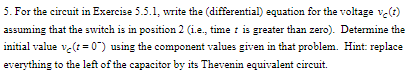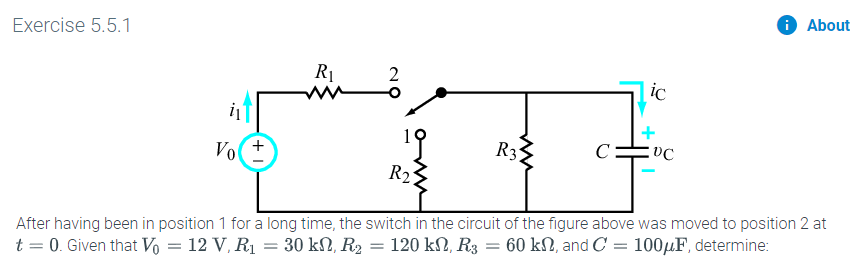# 5. For the circuit in Exercise 5.5.1, write the (differential) equation for the voltage ve(t)assuming that the switch is in position 2 (ie, time is greater than zero). Determine theinitial value v( 0") using the component values given in that problem. Hint replaceeverything to the left of the capacitor by its Thevenin equivalent circuit AboutExercise 5.5.12icR3C:VoR2After having been in position 1 for a long time, the switch in the circuit of the figure above was moved to position 2 at60 k2, and C 1004F, determine:120 kΩ, R3t 0. Given that Vo = 12 V, R1 = 30 k2, R2

Question
9 viewshelp_outlineImage Transcriptionclose5. For the circuit in Exercise 5.5.1, write the (differential) equation for the voltage ve(t) assuming that the switch is in position 2 (ie, time is greater than zero). Determine the initial value v( 0") using the component values given in that problem. Hint replace everything to the left of the capacitor by its Thevenin equivalent circuit fullscreenhelp_outlineImage TranscriptioncloseAbout Exercise 5.5.1 2 ic R3 C: Vo R2 After having been in position 1 for a long time, the switch in the circuit of the figure above was moved to position 2 at 60 k2, and C 1004F, determine: 120 kΩ, R3 t 0. Given that Vo = 12 V, R1 = 30 k2, R2 fullscreen
check_circle

Step 1

To find the initial voltage, VC (t = 0-) and differential equation of voltage, VC (t) across capacitor in the given circuit:

Step 2

Initially before time t = 0, the circuit position is connected at position 1.

The reduced circuit for time t < 0 can be drawn as:

Step 3

From the reduced circuit, As there is no voltage source present in the circuit, the voltage acro...

### Want to see the full answer?

See Solution

#### Want to see this answer and more?

Solutions are written by subject experts who are available 24/7. Questions are typically answered within 1 hour.*

See Solution
*Response times may vary by subject and question.
Tagged in

### Electrical Engineering